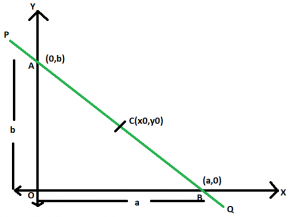GeeksforGeeks App
Open AppBrowser
Continue

# Equation of straight line passing through a given point which bisects it into two equal line segments

Given a straight line which passes through a given point (x0, y0) such that this point bisects the line segment in two equal line segments. The task is to find the equation of this straight line.
Examples:

Input: x0 = 4, y0 = 3
Output: 3x + 4y = 24
Input: x0 = 7, y0 = 12
Output: 12x + 7y = 168

Approach:Let PQ be the line and AB be the line segment between the axes. The x-intercept and y-intercept are a & b respectively.
Now, as C(x0, y0) bisects AB so,
x0 = (a + 0) / 2 i.e. a = 2x0
Similarly, y0 = (0 + b) / 2 i.e. b = 2y0
We know that the equation of a straight line in intercept form is,

x / a + y / b = 1
Here, a = 2x0 & b = 2y0
So, x / 2x0 + y / 2y0 = 1
or, x / x0 + y / y0 = 2
Therefore, x * y0 + y * x0 = 2 * x0 * y0

Below is the implementation of the above approach:

## C++

 `// C++ implementation of the approach``#include ``using` `namespace` `std;` `// Function to print the equation``// of the required line``void` `line(``double` `x0, ``double` `y0)``{``    ``double` `c = 2 * y0 * x0;``    ``cout << y0 << ``"x"``         ``<< ``" + "` `<< x0 << ``"y = "` `<< c;``}` `// Driver code``int` `main()``{``    ``double` `x0 = 4, y0 = 3;``    ``line(x0, y0);` `    ``return` `0;``}`

## Java

 `// Java implementation of the approach``class` `GFG``{``    ` `// Function to print the equation``// of the required line``static` `void` `line(``double` `x0, ``double` `y0)``{``    ``double` `c = (``int``)(``2` `* y0 * x0);``    ``System.out.println(y0 + ``"x"` `+ ``" + "` `+``                       ``x0 + ``"y = "` `+ c);``}` `// Driver code``public` `static` `void` `main(String[] args)``{``    ``double` `x0 = ``4``, y0 = ``3``;``    ``line(x0, y0);``}``}` `// This code is contributed``// by Code_Mech`

## Python3

 `# Python 3 implementation of the approach` `# Function to print the equation``# of the required line``def` `line(x0, y0):``    ``c ``=` `2` `*` `y0 ``*` `x0``    ``print``(y0, ``"x"``, ``"+"``, x0, ``"y="``, c)` `# Driver code``if` `__name__ ``=``=` `'__main__'``:``    ``x0 ``=` `4``    ``y0 ``=` `3``    ``line(x0, y0)``    ` `# This code is contributed by``# Surendra_Gangwar`

## C#

 `// C# implementation of the approach``using` `System;` `class` `GFG``{``    ` `// Function to print the equation``// of the required line``static` `void` `line(``double` `x0, ``double` `y0)``{``    ``double` `c = (``int``)(2 * y0 * x0);``    ``Console.WriteLine(y0 + ``"x"` `+ ``" + "` `+``                    ``x0 + ``"y = "` `+ c);``}` `// Driver code``public` `static` `void` `Main(String[] args)``{``    ``double` `x0 = 4, y0 = 3;``    ``line(x0, y0);``}``}` `/* This code contributed by PrinciRaj1992 */`

## PHP

 ``

## Javascript

 ``

Output

`3x + 4y = 24`

Time Complexity: O(1)
Auxiliary Space: O(1)

My Personal Notes arrow_drop_up